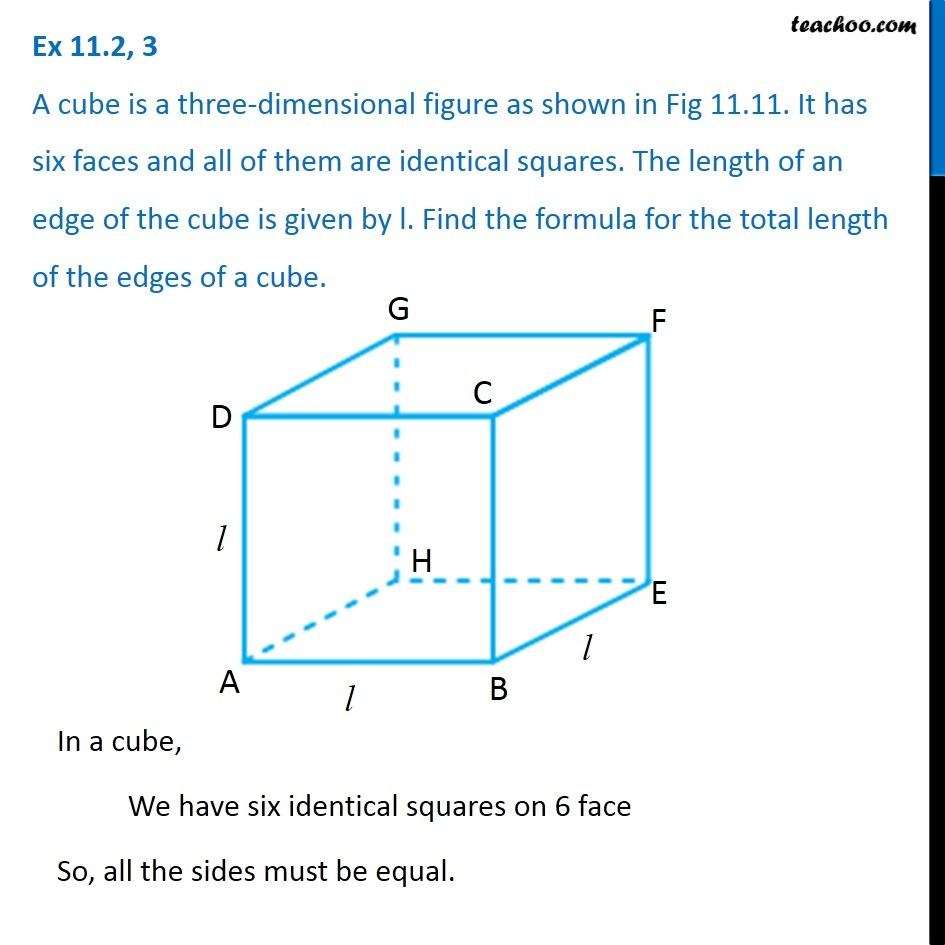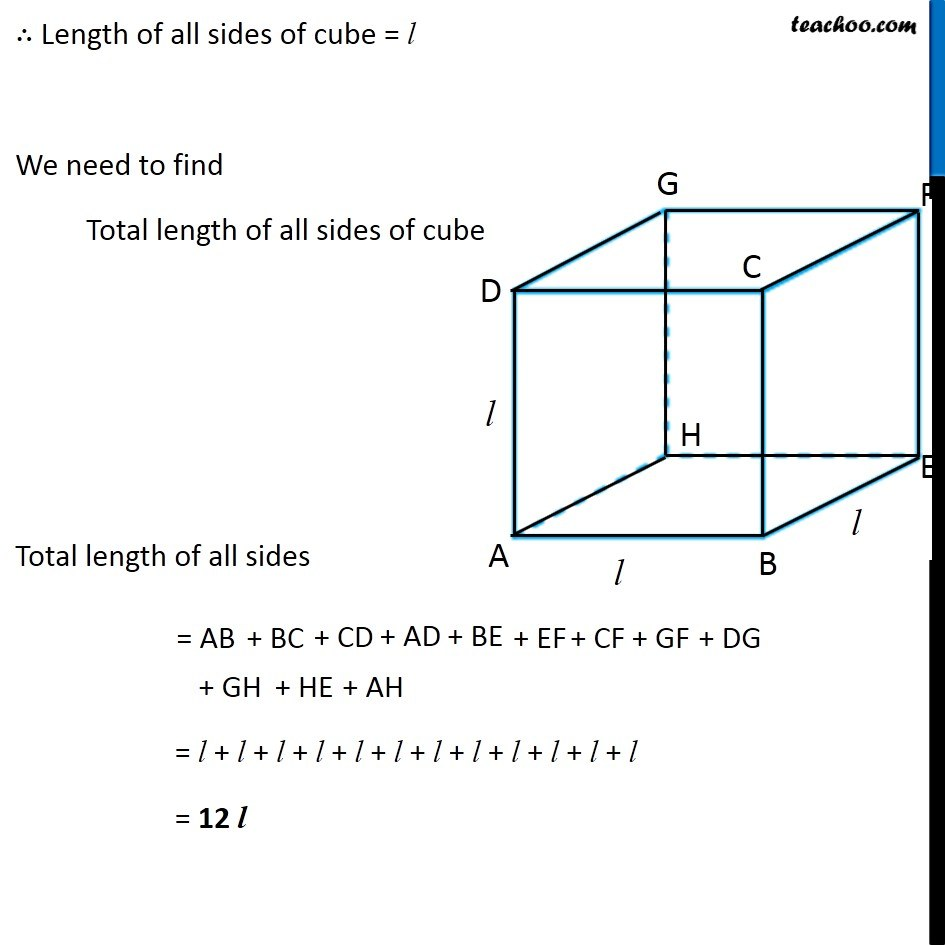Perimeter as variable

Chapter 11 Class 6 Algebra
Concept wiseLearn in your speed, with individual attention - Teachoo Maths 1-on-1 Class

### Transcript

Question 3 A cube is a three-dimensional figure as shown in Fig 11.11. It has six faces and all of them are identical squares. The length of an edge of the cube is given by l. Find the formula for the total length of the edges of a cube. In a cube, We have six identical squares on 6 face So, all the sides must be equal. ∴ Length of all sides of cube = l We need to find Total length of all sides of cube Total length of all sides = l + l + l + l + l + l + l + l + l + l + l + l = 12 l